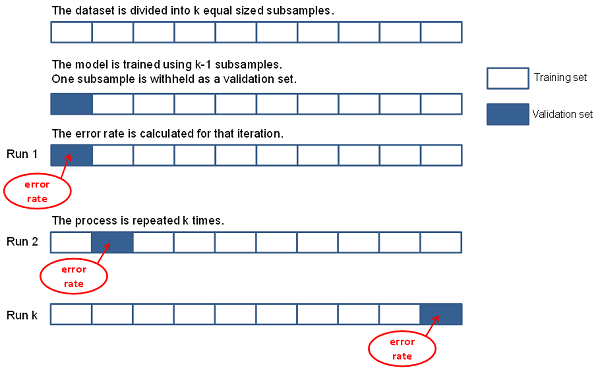# 1.1 - 8.10 - CrossValidation (ML Engine) - Teradata Vantage

## Teradata Vantage™ - Machine Learning Engine Analytic Function Reference

Product
Release Number
1.1
8.10
Published
October 2019
Content Type
Programming Reference
Publication ID
B700-4003-079K
Language
English (United States)

Cross-validation (or rotation estimation) is a model-validation technique for assessing how the results of a statistical analysis generalize to an independent data set. Use this technique when your goal is prediction, to estimate how accurately a predictive model performs in practice.

Typically, you train a model on a training set (a data set for which you know the response variable) and validate the model on a test set or validation set (a different data set for which you know the response variable). The CrossValidation function lets you have multiple test sets by partitioning the training set. The function can thus provide insight into how a model might generalize to an independent data set.

The CrossValidation function works as follows:
1. It partitions the data randomly into k equal-sized subsamples.
2. It keeps one group as a test set and trains the model on the rest of the data.
3. It uses the trained model on the test set and calculates the error rate.
4. It repeats the preceding steps k times, using each of the k subsamples as the test set.
K-Fold Cross-Validation